Oliver Knill Talk given October 19, 2000 at Montana State University Bozeman

# Some intriguing open problems in Hamiltonian dynamics

 This is a personal list of problems, I currently like to think about (Oct. 2000). Except of 5),8),10),13) (to which I don't know whether they have been asked before), these are "old" problems. Statements after GUESS: answer chosen when I would be forced to give an opinion. Statements after SOURCE: a starting point in the literature. Clicking on a thumbnail picture reveals the corresponding transparency. Image source to 9) http://turnbull.dcs.st-and.ac.uk/history. Image source to 2) NASA. The other transparencies were made using Mathematica 4.0 and the raytracing software Povray 3.0 both running on the Linux operating system.1) UNSTABLE EXTERIOR BILLIARDS. Is there a convex table in the plane such that the exterior billiard map T at this table has an unstable orbit (i.e. the set |Tn(x,y)| is unbounded). KNOWN: Stability for smooth tables by KAM and classes of polygons (Vivaldi-Shaidenko). GUESS: All convex tables are stable. If this is wrong and a table with an unstable example should exist, the table should be obtained by a Baire category argument. SOURCE: Problem posed by Hammer in 1960. See J. Moser "Stable and random Motion in dynamical systems", 1973, J. Moser, "Is the solar system stable", 1978., Tabachnikov, "Billiards", 1995 SLIDE: Shows some orbits of the exterior billiard in the case of a realanalytic table. Orbits computed with Mathematica.2) N-BODY PROBLEM: What is the measure of the set of initial conditions of the Newtonian N-body problem leading to global solutions? The complement, the set of singularities splits into collision and non-collision singularities. KNOWN: Collision singularities have measure zero (Saari). Non-collision singularities exist for N>4 particles (Xia). In 4 dimensions, collisions can have positive measure. Almost everywhere existence for N=3,4 particles known (Saari). Smooth mass distributions (Vlasov generalization of n-body problem) leads to global solutions (Pfaffelmoser 1992). GUESS: can an almost everywhere existence result be obtained by extending the Vlasov existence theorem to a BBGKY hierarchy? SOURCE: Probably first posed by Painlevé or Poincaré. The problem appears in the list of B.Simon: "Fifteen problems in mathematical physics", 1984 as Problem 1A. For Vlasov, see R.T. Glassey "The Cauchy problem in kinetic theory". SLIDE: Shows colliding galxies Picture Source: Nasa.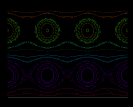3) BIRKHOFF BILLIARDS: Is there a smooth Birkhoff billiard (a billiard in convex table) for which the dynamics has positive entropy? The entropy is by Pesin given by the averaged Lyapunov exponent limn n-1 log E[ ||d Tn|| ], where dTn is the Jacobean of the n'th iterate Tn of the billiard map T. KNOWN: Non-smooth examples like the Bunimovich stadium are known. Smooth, strictly convex billiards are not ergodic. (KAM leads to "whisper-galleries" near the boundary). GUESS: Most smooth Birkhoff billiards should have positive entropy. Positive entropy measured for example for the table x4+y4=1. SOURCE: Problem probably first posed by Y. Sinai. See S. Tabachnikov, "Billiards", 1995. For entropy measurements, see for example M. Jeng and O. Knill, "Billiards in the lp unit balls of the plane", 1996 SLIDE: Shows some orbits of a smooth billiard map. Orbits computed with Mathematica.4) BIRKHOFF-PORITSKI CONJECTURE. Is every integrable Birkhoff billiard an ellipse? Related question: if a Birkhoff billiard map T is conjugated to an algebraic map, is the table then an ellipse. KNOWN: Problem depends on the definition of integrability. One possible definition: every ergodic invariant measure leads to a dynamical system with discrete spectrum. Under a strong integrability condition (foliation of the phase space by invariant circles), the table must be a circle (Bialy). A real-analytic table with integral which is analytic in the angle must be an ellipse. GUESS: There might be counter examples of tables of constant width (de Verdière) and these billiard maps are non-algebraic. SOURCE: Problem posed by H. Poritsky, Annals of Math, 51, 1950. Unclear whether it is a question of Birkhoff. SLIDE: Shows an orbit of a billiard in a table of equal width. The orbit is located on an invariant curve. Integrability would mean that every orbit behaves in an almost periodic way. Computed with Mathematica.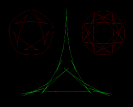5) FRACTAL CAUSTICS. Are there geodesic flows or Birkhoff billiards with fractal caustics? KNOWN: Examples of tables with nowhere differentiable caustics are known (tables of equal width). GUESS: For any s bigger or equal to 1 and smaller than 2, there is a caustic of a convex billiard with Hausdorff dimension s. For any s bigger or equal than 1, there exists a Riemannian manifold M nd a point such that the caustic of this point has Hausdorff dimension s. SOURCE: O. Knill, "On non-convex caustics of convex billiards", 1998 SLIDE: Green: Caustic which is nowhere differentiable.6) CONJUGACY. If T1=ST2S-1 with a homeomorphism S then the tables are similar. A related question is the Kac inverse problem: "Can one hear the shape of a convex drum?" KNOWN: The shape of non-convex tables can not be heard because there are isospectral nonconvex tables (Gordon-Webb following Buser,Sundada) GUESS: One should be able to hear the shape of a real-analytic convex drum. Conjugacy of two Birkhoff billiard maps should imply similarity of such tables. SOURCE: The billiard conjugacy problem was posed by V.Guillemin in the late seventies. SLIDE: Periodic orbit of a billiard near a periodic orbit of period 3. If this table were conjugated to an other table, there would again exist an orbit of this type.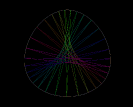7) PERIODIC ORBITS. a) The set of n-periodic orbits of a smooth strictly convex Birkhof billiard is nowhere dense for all n. b) Does every polygonal Birkhoff billiard have a periodic orbit? KNOWN: Yes, for n=2,3,4, periodic orbits are nowhere dense (Rychlik). Polygons with angles which are a rational multiply of Pi have periodic orbits (actually a generic orbit is periodic (Boshernitzan-Stepin)). GUESS: a) All n-periodic orbits are nowhere dense. b) All polygonal Birkhoff billiards should have periodic orbits. SOURCE: S. Tabachnikov, "Billiards", 1995 SLIDE: Orbit of period 31.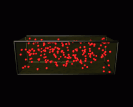8) FREE GAS IN MOVING CONTAINER. Does a free gas coupled to a convex rigid container by the law of momentum conservation converge weakly to an equilibrium and the container (which only moves by translation) come to rest? KNOWN. The dynamics exists. For a discrete mass distribution, the Hamiltonian system is finite dimensional and there is Poincaré recurrence. GUESS. For initial gas and phase space densities near equilibrium in one dimensions the boundary comes to rest like 1/t. In general, it should depend on the dynamical properties of the billiard obtained when the container is at rest. i.e. exponential convergence with strong mixing properties of the billiard would imply exponential fast convergence to equilibrium. SOURCE. O. Knill, "An existence theorem for Vlasov dynamics with moving boundaries. MP-ARC document 00-38 SLIDE: A free gas in a container. Each particle hitting the boundary moves the boundary. While the system is integrable if the boundary is kept fixed, particles are coupled through the boundary when the container can move. Picture rendered in Povray 3.0.9) KOLMOGOROV PROBLEM. Is there a Hamiltonian system with a smooth invariant torus, on which the induced dynamics is mixing? KNOWN: Situations with weakly mixing tori. No mixing can happen on two-dimensional tori. GUESS. Yes, there should exist examples in higher (d>3) dimensions. SOURCE. According to Arnold, this is a question of Kolmogorov which motivated KAM theory. (See O. Knill, Weakly mixing invariant tori of Hamiltonian systems, 1999) SLIDE: Kolmogorov. Picture taken from Mac Tutor.10) THE GOOD THE BAD AND THE UGLY. Call the "good set" the maximal invariant subset in the phase space of a Hamiltonian systems for which the invariant Liouville measure is almost periodic. Call the "bad set" the Pesin set on which the invariant measure has a positive Lyapunov exponent. Call the "ugly set" the complement of the union of these two sets. Is there an example where the ugly set has positive measure (that is, where it is physically relevant)? KNOWN. The GOOD SET has often positive measure (KAM). The bad set is believed to have positive measure in many cases. GUESS: The ugly set has positive measure too in general and the dynamics on this set has some weak mixing properties on sets of positive measure. SOURCE: O. Knill, Singular continuous spectrum and quantitative rates of weakly mixing, 1998 SLIDE: Shows the Fourier transform of the equilibrium measure of the Cantor set, a case already studied by N. Wiener.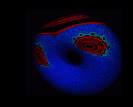11) MANÉ'S LAST THEOREM: In the set of area-preserving C1 diffeomorphisms on a compact manifold, one has generically hyperbolicity or zero Lyapunov exponents? KNOWN. The hyperbolic diffeomorphisms form an open set. Away from diffeomorphisms with hyperbolicity, one generically has a dense set of elliptic orbits (Newhouse). Yes, for dim=2. (preprint of J. Bochi, Sept. 15, 2000). GUESS. Away from Anosov maps, there exists a dense set of Newhouse maps: diffeomorphis having a set of periodic orbits with full measure. This would imply Mané's last theorem and also show that generically, an area preserving non-Anosov C1 map has a Koopman operator with singular continuous spectrum. SOURCE. R. Mané, "The Lyapunov exponents of a generic area-preserving diffeomorphism, 1995". SLIDE: Smooth maps seem to contradict Man\'e's last theorem. But smooth maps like here the Standard map form a very thin set in the measure preserving C1 diffeos.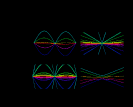13) CALOGERO-MOSER-VLASOV. The Calogero-Moser system is a n-body problem on the real line, where particles interact with the inverse square potential. The question is whether in infinite-dimensions the dynamics exists. As for any infinite-dimensional integrable system, the question arrizes whether it is integrable in the sense that every invariant measure gives rize to almost periodic dynamics. KNOWN. There is an existence proof for some continuous measures located on one-dimensional sets (at each point a particle has a specific speed). GUESS. Yes, the dynamics should exist for any smooth initial measure in the phase space and the motion is conjugated to an isospectral deformation of a Calderon-Zygmund operator. SOURCE. J. Moser, "Three Integrable Hamiltonian Systems Connected with Isospectral Deformations". SLIDE: Some orbits of the finite dimensional Calogero-Moser system. The orbits have been computed in Mathematica by giving explicit formulas.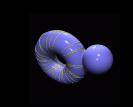14) MATHER THEORY NEAR INTEGRABLE SYSTEMS. Are there quasiperiodic global minimals for metrics on the torus which are close to a flat three dimensional torus? (A geodesic is a global minimal if for any two points on the geodesic, the piece between them is a minimal solution.) KNOWN. Not necessarily for metrics far away from integrable situations (Hedlund examples). Yes in two-dimensions (Mather theory). GUESS: Beside the KAM transition, there should be a transition, where Mather theory breaks down. SOURCE: "J. Moser, Selected topics in the calculus of variations, ETH, 1988". SLIDE: A piece of a geodesic orbit on a torus.
Back to Oliver's Homepage